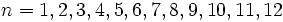# Number of groups of given order

This article defines an arithmetic function or number-theoretic function: a function from the natural numbers to a ring (usually, the ring of integers, rational numbers, real numbers, or complex numbers).
View a complete list of arithmetic functions

## Definition

Letbe a natural number. The number of groups of orderis the number of isomorphism classes of groups whose order is. In other words, it is the maixmum possible number of pairwise non-isomorphic groups of order.

## Behavior

The ID of the sequence in the Online Encyclopedia of Integer Sequences is A000001

### Initial values

Forthe number of groups is.

### Lower bound

The numbersfor which there is only one isomorphism class of groups of orderare termed cyclicity-forcing numbers. These are also the only orders of groups whose automorphism group has coprime order to the group itself. Further information: Groupprops:Classification of cyclicity-forcing numbers, Groupprops:Coprime automorphism group implies cyclic with order a cyclicity-forcing number

A number is a cyclicity-forcing number if and only if it is a product of distinct primes such that nodividesfor prime divisors. In particular, all prime numbers are cyclicity-forcing, and thus, the number of groups function takes the valuefor infinitely many natural numbers. Note that there are composite cyclicity-forcing numbers, the smallest of which is.

### Upper bound

High values are typically attained at prime powers.